﻿ 视觉感知式场景文字检测定位方法
«上一篇文章快速检索 高级检索

 智能系统学报2017, Vol. 12Issue (4): 563-569  DOI: 10.11992/tis.2016040110

### 引用本文LYU Guoning, GAO Min. Scene text detection and localization scheme with visual perception mechanism[J]. CAAI Transactions on Intelligent Systems, 2017, 12(4): 563-569. DOI: 10.11992/tis.201604011.### 文章历史

1. 郑州师范学院 网络管理中心, 河南 郑州 450044;
2. 郑州师范学院 信息科学与技术学院, 河南 郑州 450044

Scene text detection and localization scheme with visual perception mechanism
LYU Guoning1, GAO Min2
1. Network Management Center, Zheng Zhou Normal University, Zheng Zhou 450044, China;
2. School of Information Science and Technique, Zheng Zhou Normal University, Zheng Zhou 450044, China
Abstract: To solve the detection problem with respect to the interference of complex backgrounds in natural scenes, in this paper, we propose a scene text detection and localization scheme based on a visual perception mechanism. The human visual perception mechanism is commonly divided into the fast parallel pre-attention step and the slow serial attention step. In our proposed scheme, we first precedes the pre-attention step with two visual saliency methods and then implement the attention step using a stroke feature and the relationship between characters. Our experimental results show the scheme to be competitive with respect to the ICDAR 2013 and the scene Chinese-character dataset. It is also suitable for English and Chinese character detection of natural scenes under complex background conditions.
Key words: visual perception    visual saliency    swt    scene text    text detection and localization    visual attention    Chinese text    English text

1 视觉显著性算法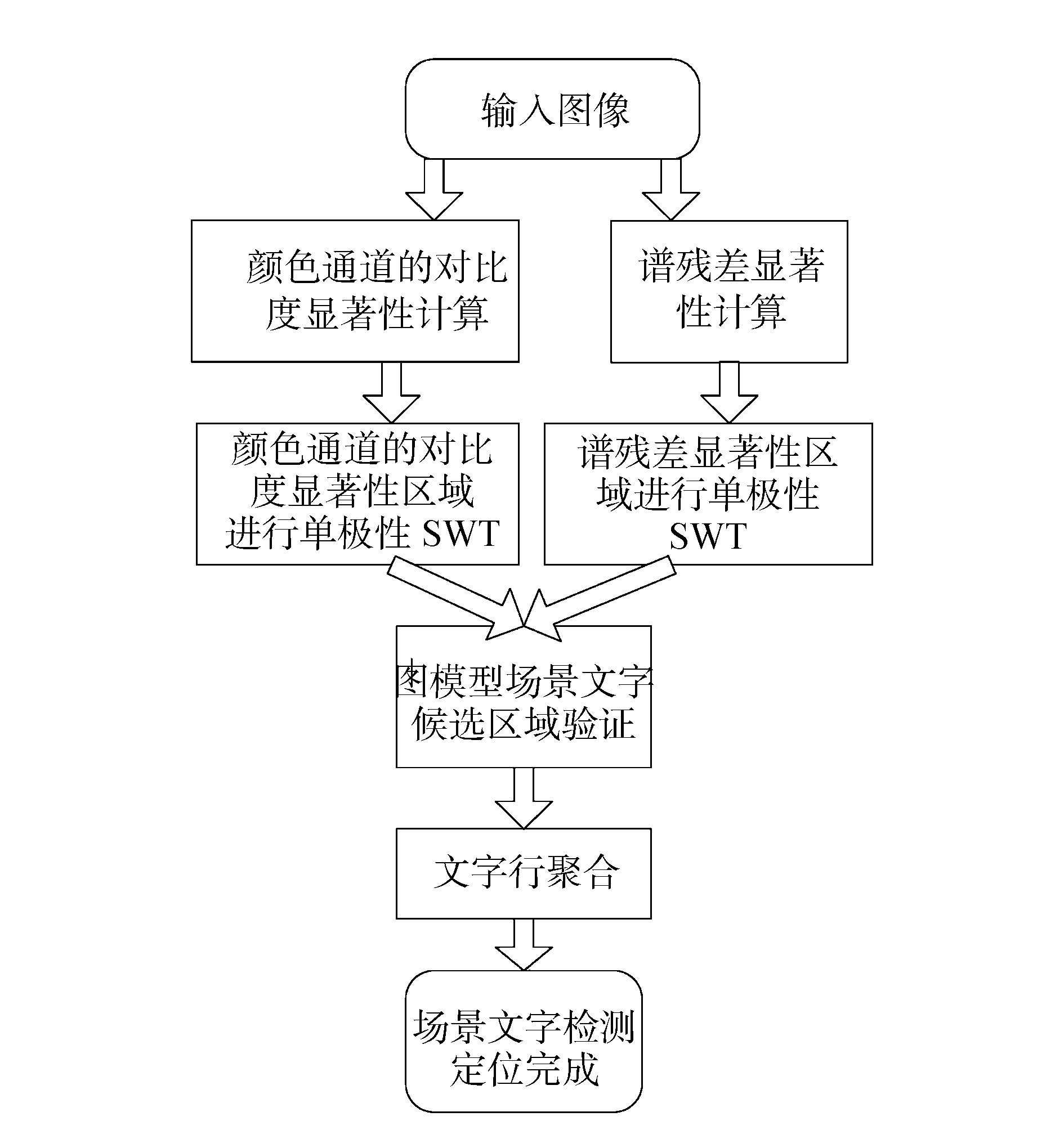图 1 算法流程图 Fig.1 Algorithm flow chart
1.1 颜色通道的对比度视觉显著性模型

 $\begin{array}{c} L=\frac{r+g+b} 3\\\text{RG}=\frac{r－g} {\max(r,g,b)}\\\text{BY}=\frac{b－\min(r,g)} {\max(r,g,b)} \end{array}$ (1)

 $C(i,j)=\text{abs}(I(i,j)－\bar I(i,j))$ (2)
 $ws=(1/2^σ)×\min(w,h)$ (3)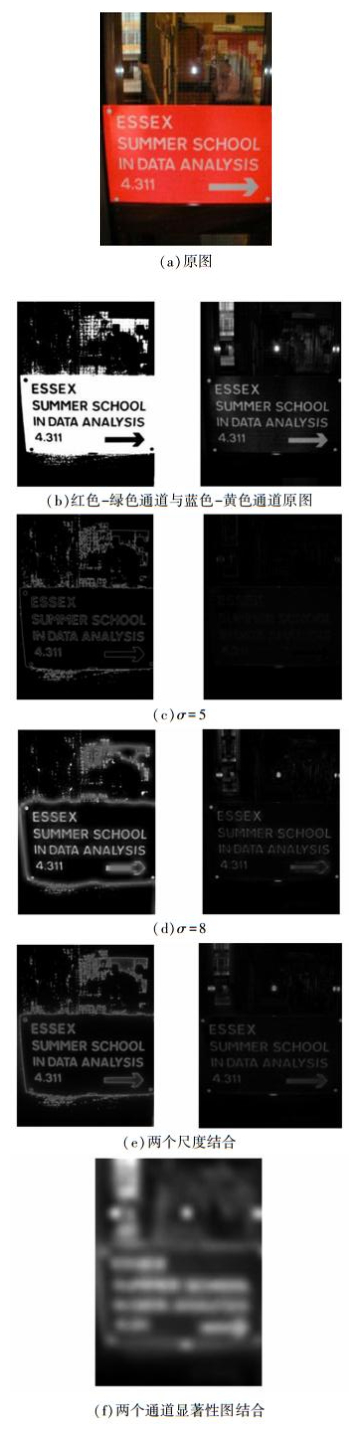（a）原图（b）红色-绿色通道与蓝色-黄色通道原图（c）σ=5（d）σ=8（e）两个尺度结合（f）两个通道显著性图结合 图 2 颜色通道的显著性效果图 Fig.2 Saliency map of color channel
1.2 谱残差视觉显著性模型

 $S=\log((A(I))－h(I)*\log(A(I))$ (4)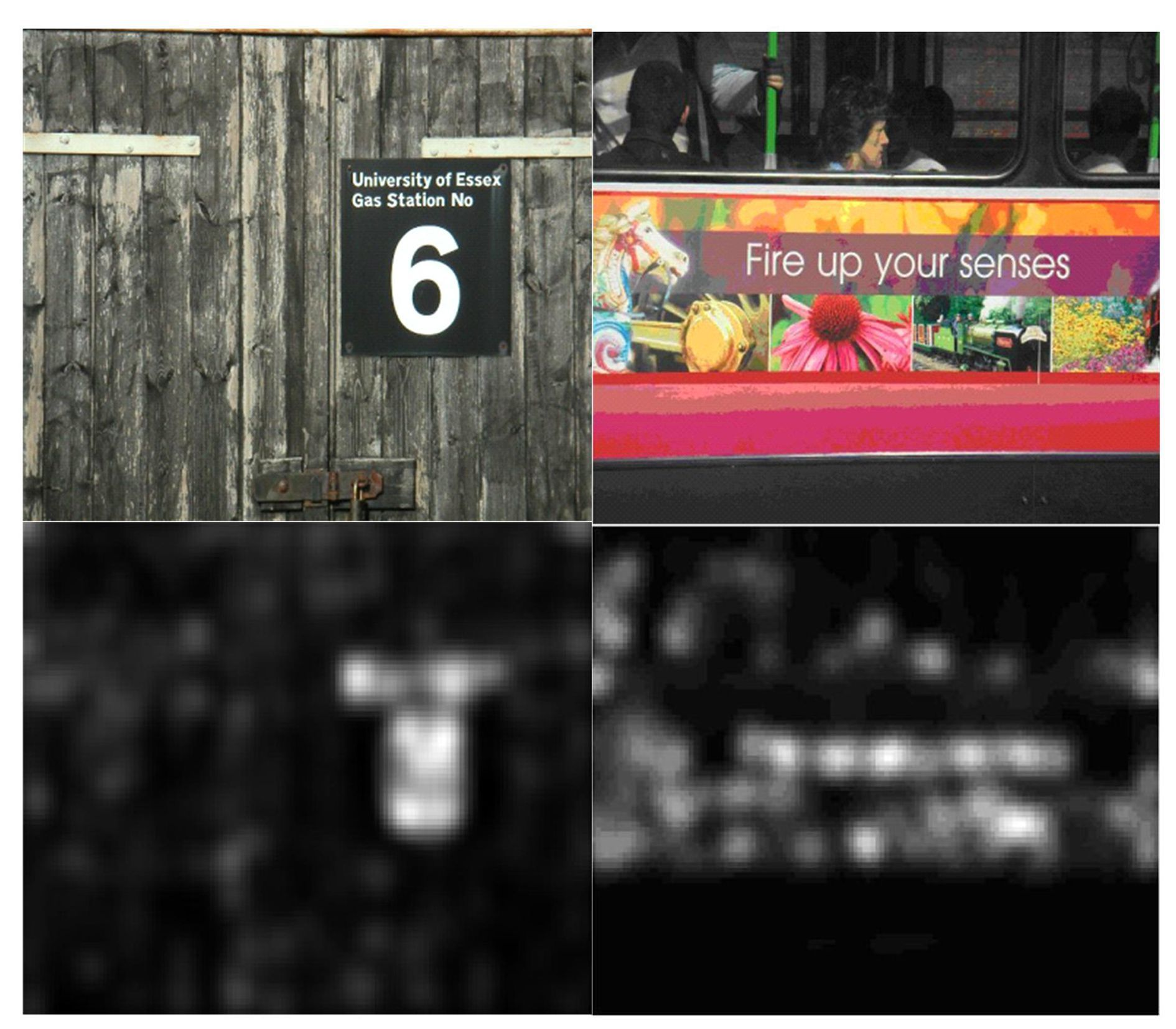图 3 谱残差显著性效果图 Fig.3 Spectral residue saliency map
1.3 显著性区域

1）首先采用大津法得到阈值t，然后在训练数据集中设定显著区域中文字召回率的阈值$T_R$，初始化系数$α$为1，以0.01为步长递减系数$α$，直到首次显著区域中文字召回率R首次达到阈值${T}_{R}$即停止，最终通过式(5) 计算得到阈值${T′}_{s}$。颜色通道的对比度显著性算法系数为${α}_{c}=1$，谱残差显著性算法系数为${α}_{s}=0.73$。在得到两种显著性区域后，分别进行数学形态学操作，并填补去除显著性区域中的孔洞。

 $T′_s=α×t$ (5)
2 单极性笔画宽度变换算法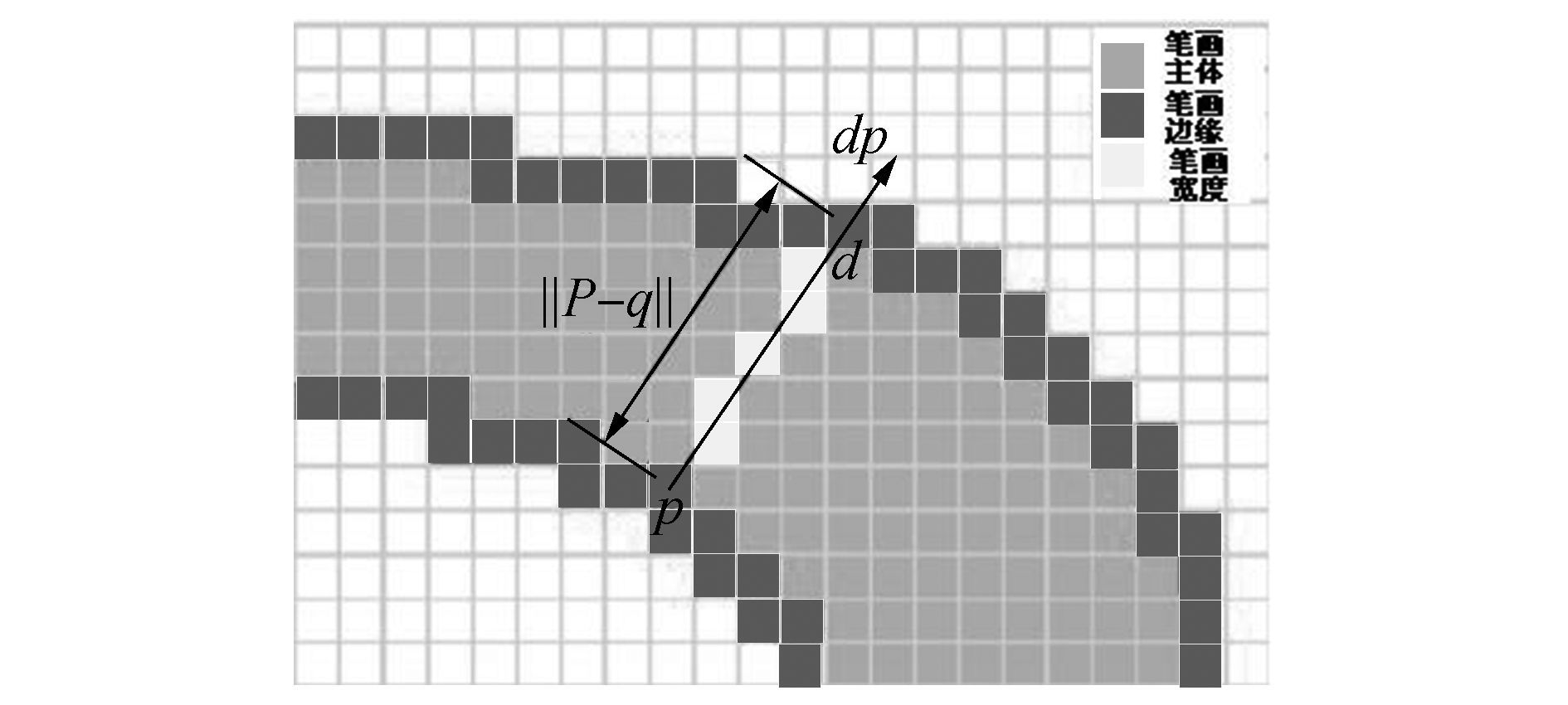图 4 笔画宽度计算方法图 Fig.4 Stroke width map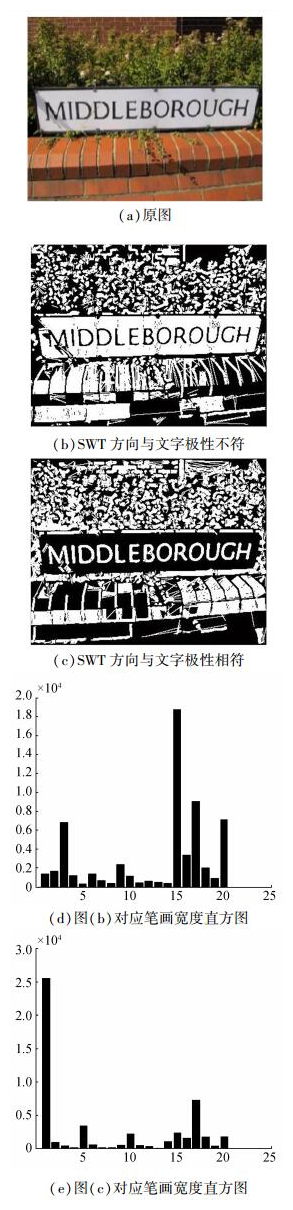图 5 场景文字极性与笔画宽度直方图关系 Fig.5 The relation between the pole of scene text and stroke width histogram

① 起始阶段不做极性判断，任意选择一种极性在显著性区域进行SWT。若其间，任一边缘像素的射线越过显著性区域边界，则此显著性区域为相反极性。

② 如果两种极性SWT计算中均未发生边缘像素的射线越过显著性区域边界情况，则对该显著性区域两种极性的笔画宽度图求直方图。按照式(6) 计算两种极性的笔画宽度直方图的集中度，集中度较大的极性为此显著性区域的极性。

 $f(h)=\frac1 N\sum\limits^N _{i=2}(h(i)－h(i－1))$ (6)

3 基于图模型的文字候选区域验证与文字行聚合

 $\begin{array}{c} \text{dist}(x_i,x_j)<2×\min(\max(w_i,h_i),\max(w_j,h_{j}))\\ ∧\min(w_{i,}w_j)/\max(w_i,w_j)>0.4\\ ∧\min(h_{i,}h_j)/\max(h_i,h_j)>0.4 \end{array}$ (7)

 $E(A)=\sum\limits^P _{p=1}U_p(A)+\sum\limits_{\{p,q\}∈N}B_{\{p,q\}}(A)$ (8)

 $B_{\{p,q\}}=\exp\left(－\frac{0.5×{\text{Dis}}_{\text{color}}+0.5×{\text{Dis}}_{\text{stroke}}} {2×σ^2}\right)$ (9)表 1 图模型用到的特征 Tab.1 The feature used in graph model

4 实验与分析表 2 ICDAR 2013文字定位竞赛数据集实验结果 Tab.2 The result in ICDAR 2013 Task2 dataset表 3 场景汉字数据集实验结果 Tab.3 The result in Chinese scene text dataset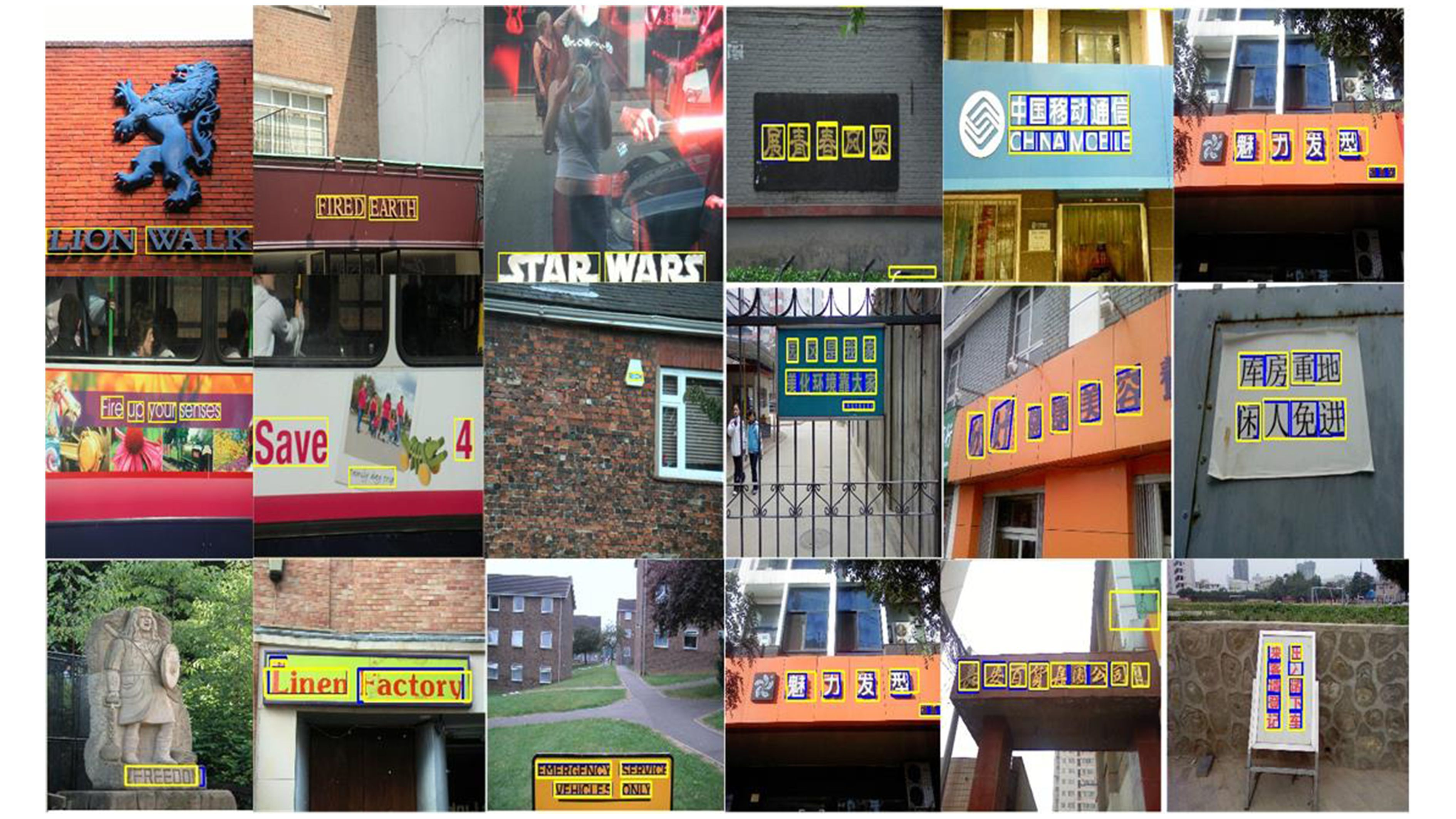图 6 算法效果图 Fig.6 Algorithm result
5 结论与展望

  JUNG K, KIM K I, JAIN A K. Text information extraction in images and video:a survey[J]. Pattern recognition, 2004, 37(5): 977-997. DOI:10.1016/j.patcog.2003.10.012 (0)  BAI Bo, YIN Fei, LIU Chenglin. Scene text localization using gradient local correlation[C]//International Conference on Document Analysis and Recognition, Washington DC, 2013:1412-1416. (0)  姜维, 卢朝阳, 李静, 等. 针对场景文字的基于视觉显著性和提升框架的背景抑制方法[J]. 电子与信息学报, 2014, 36(3): 617-623. JIANG Wei, LU Zhaoyang, LI Jing, et al. Visual saliency and boosting based background suppression for scene text[J]. Journal of electronics & information technology, 2014, 36(3): 617-623. (0)  CONG Yao, et al. Detecting texts of arbitrary orientations in natural images[C]//IEEE Conference on Computer Vision and Pattern Recognition, Providence. 2012:1083-1090. (0)  LI Yao, JIA Wenjing, SHEN Chunhua, et al. Characterness:an indicator of text in the wild[J]. IEEE transactions on image processing, 2014, 23(4): 1666-1677. DOI:10.1109/TIP.2014.2302896 (0)  赵春晖, 王佳, 王玉磊. 采用背景抑制和自适应阈值分割的高光谱异常目标检测[J]. 哈尔滨工程大学学报, 2016, 37(2): 278-283. ZHAO Chunhui, WANG Jia, WANG Yulei. Hyperspectral anomaly detection based on background suppression and adaptive threshold segmentation[J]. Journal of Harbin engineering university, 2016, 37(2): 278-283. (0)  HOU X D, ZHANG L Q. Saliency detection:a spectral residual approach[C]//IEEE Conference on Computer Vision and Pattern Recognition, Minneapolis, 2007:1-8. (0)  EPSHTEIN B, OFEK E, WEXLER Y. Detecting text in natural scenes with stroke width transform[C]//IEEE International Conference on Computer Vision and Pattern Recognition. San Francisco, 2010:2963-2970. (0)  BOYKOV Y, KOLMOGOROV V. An experimental comparison of min-cut/max-flow algorithms for energy minimization in vision[J]. IEEE transaction pattern analysis and machine intelligence, 2004, 26(9): 1124-1137. DOI:10.1109/TPAMI.2004.60 (0)  KARATZAS D, SHAFAIT F, UCHIDA S, et al. ICDAR 2013 Robust Reading Competition[C]//IEEE International Conference on Document Analysis and Recognition. Washington DC, 2013:1484-1493. (0)  LUCAS S M. ICDAR 2005 text locating competition results[C]//8th International Conference on Document Analysis and Recognition. 2005:80-84. (0)  姜维, 卢朝阳, 李静, 等. 基于角点类别特征和边缘幅值方向梯度直方图统计特征的复杂场景文字定位算法[J]. 吉林大学学报:工学版, 2013, 43(1): 250-255. JIANG Wei, LU Zhaoyang, LI Jing, et al. Text localization algorithm in complex scene based on corner-type feature and histogram of oriented gradients of edge magnitude statistical feature[J]. Journal of Jilin University:engineering and technology edition, 2013, 43(1): 250-255. (0)